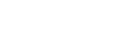The variance inflation factor (VIF) quantifies the extent of correlation between one predictor and the other predictors in a model. It is used for diagnosing collinearity/multicollinearity. Higher values signify that it is difficult to impossible to assess accurately the contribution of predictors to a model.

## How the VIF is computed

The standard error of an estimate in a linear regression is determined by four things:

• The overall amount of noise (error). The more noise in the data, the higher the standard error.
• The variance of the associated predictor variable. The greater the variance of a predictor, the smaller the standard error (this is a scale effect).
• The sampling mechanism used to obtain the data. For example, the smaller the sample size with a simple random sample, the bigger the standard error.
• The extent to which a predictor is correlated with the other predictors in a model.

The extent to which a predictor is correlated with the other predictor variables in a linear regression can be quantified as the R-squared statistic of the regression where the predictor of interest is predicted by all the other predictor variables ( ). The variance inflation for a variable is then computed as:Some statistical software use tolerance instead of VIF, where tolerance is:The VIF can be applied to any type of predictive model (e.g., CART, or deep learning). A generalized version of the VIF, called the GVIF, exists for testing sets of predictor variables and generalized linear models.

## How to interpret the VIF

A VIF can be computed for each predictor in a predictive model. A value of 1 means that the predictor is not correlated with other variables. The higher the value, the greater the correlation of the variable with other variables. Values of more than 4 or 5 are sometimes regarded as being moderate to high, with values of 10 or more being regarded as very high. These numbers are just rules of thumb; in some contexts a VIF of 2 could be a great problem (e.g., if estimating price elasticity), whereas in straightforward predictive applications very high VIFs may be unproblematic.

If one variable has a high VIF it means that other variables must also have high VIFs. In the simplest case, two variables will be highly correlated, and each will have the same high VIF.

Where a VIF is high, it makes it difficult to disentangle the relative importance of predictors in a model, particularly if the standard errors are regarded as being large. This is particularly problematic in two scenarios, where:

1. The focus of the model is on making inferences regarding the relative importance of the predictors.
2. The model is to be used to make predictions in a different data set, in which the correlations may be different.

The higher the VIF, the more the standard error is inflated, and the larger the confidence interval and the smaller the chance that a coefficient is determined to be statistically significant.

## Remedies for VIF

Where VIF is regarded as being too high for variables, the solutions are to:

• Obtain more data, so as to reduce the standard errors.
• Use techniques designed to work better with high VIFs, such as Shapley regression (note that such techniques do not actually solve the VIF problem but instead ensure that the estimates are more reliable -- i.e., consistent).
• Obtain better data, where the predictors are less correlated (e.g., by conducting an experiment)
• Recode the predictors in a way that reduces correlations (e.g., using orthogonal polynomials instead of polynomials).